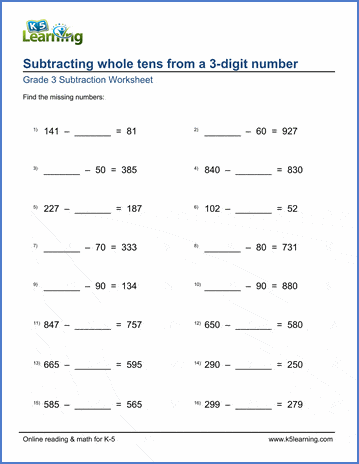# Subtract whole tens from 3-digit numbers

## Worksheets: Subtracting whole tens from 3-digit numbers with missing minuend or subtrahend

Below are six versions of our grade 3 math worksheet on subtracting whole tens (10, 20, 30 ....) from 3-digit numbers (100-999) with missing numbers in the equation. Good mental math practice! These worksheets are pdf files.## More subtraction worksheets

Explore all of our subtraction worksheets, from subtracting by counting objects to subtracting large numbers in columns.

## What is K5?

K5 Learning offers reading and math worksheets, workbooks and an online reading and math program for kids in kindergarten to grade 5.  We help your children build good study habits and excel in school.